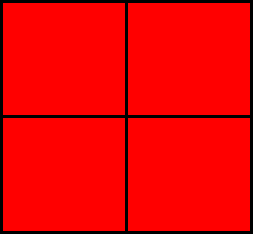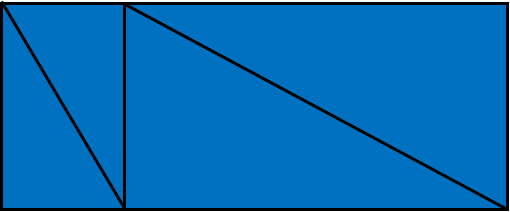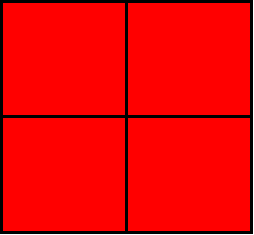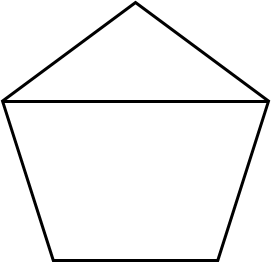1
visibility

Choose the fraction model which shows equal parts.

• A• B• CThe correct answer is.

Solution:

We consider all the fraction models one by one in order to identify the fraction having equal parts.

• We consider the first fraction model.The given fraction model has four parts.
The parts of the fraction model are the same in size. So, the fraction model shows equal parts.

• Looking at the second fraction model.The given fraction model has four parts.
The parts of the fraction model are not the same in size. So, the fraction model does not show equal parts.

• Now, we look at the last fraction model.The given fraction model has two parts.
The parts of the fraction model are not the same in size. So, the fraction model does not show equal parts.

Therefore, the fraction modelshows equal parts.